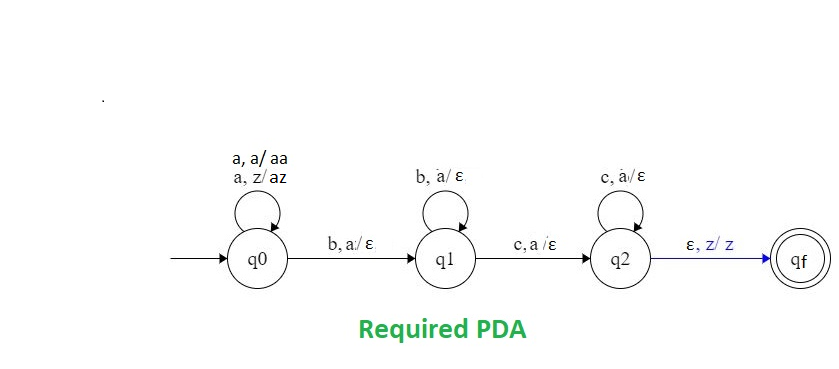# NPDA for accepting the language L = {a(m+n)bmcn | m,n ≥ 1}

• Last Updated : 02 Apr, 2019

Prerequisite – Pushdown automata, Pushdown automata acceptance by final state
Problem – Design a non deterministic PDA for accepting the languageThe strings of given language will be:

L = {aabc, aaabcc, aaabbc, aaaabbcc, ......}

In each of the string, the total sum of the number of ‘b’ and ‘c’ is equal to the number of ‘a’s. And all c’s are come after ‘a’ and ‘b’.

Attention reader! Don’t stop learning now.  Practice GATE exam well before the actual exam with the subject-wise and overall quizzes available in GATE Test Series Course.

Learn all GATE CS concepts with Free Live Classes on our youtube channel.

Explanation –
Here, we need to maintain the order of a’s, b’s and c’s. That is, all the a’s are are coming first and then all the b’s are coming after that all the c’s. Thus, we need a stack along with the state diagram. The count of a’s, b’s and c’s is maintained by the stack. We will take 2 stack alphabets:= { a, z }

Where,= set of all the stack alphabet
z = stack start symbol.

Approach used in the construction of PDA –
As we want to design a NPDA, thus every time ‘a’ comes before ‘b’and ‘b’ comes before ‘c’.First we have to count number of a’s and that number should be equal to number of b’s. When all b’s are finished, then count number of a’s and that should be equal to number of c’s.

For all the ‘a’ we will push ‘a’ into the stack each time and then start popping them when ‘b’s are coming. After finishing the popping by ‘b’s and after ‘c’s are coming we will pop these ‘a’ from the stack each time. So, at the end if the stack becomes empty then we can say that the string is accepted by the PDA.

Stack transition functions –(q0, a, z)(q0, az)(q0, a, a)(q0, aa)(q0, b, a)(q1,)(q1, b, a)(q1,)(q1, c, a)(q2,)(q2, c, a)(q2,)(q2,,z)(qf, z)


Where, q0 = Initial state
qf = Final state= indicates pop operation.
State transition diagramNote: This language is similar to the language, but we useinstead of.

My Personal Notes arrow_drop_up